Questions - Forming equations and solving

Chapter 12 Class 12 Linear Programming
Serial order wise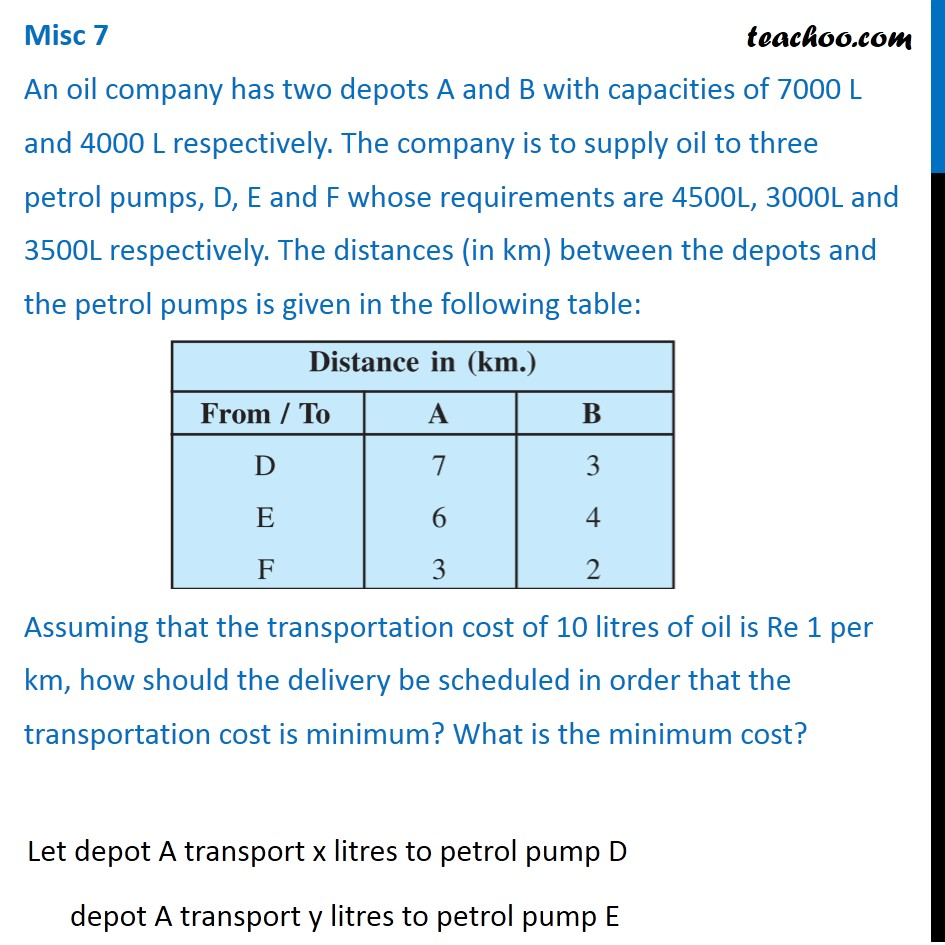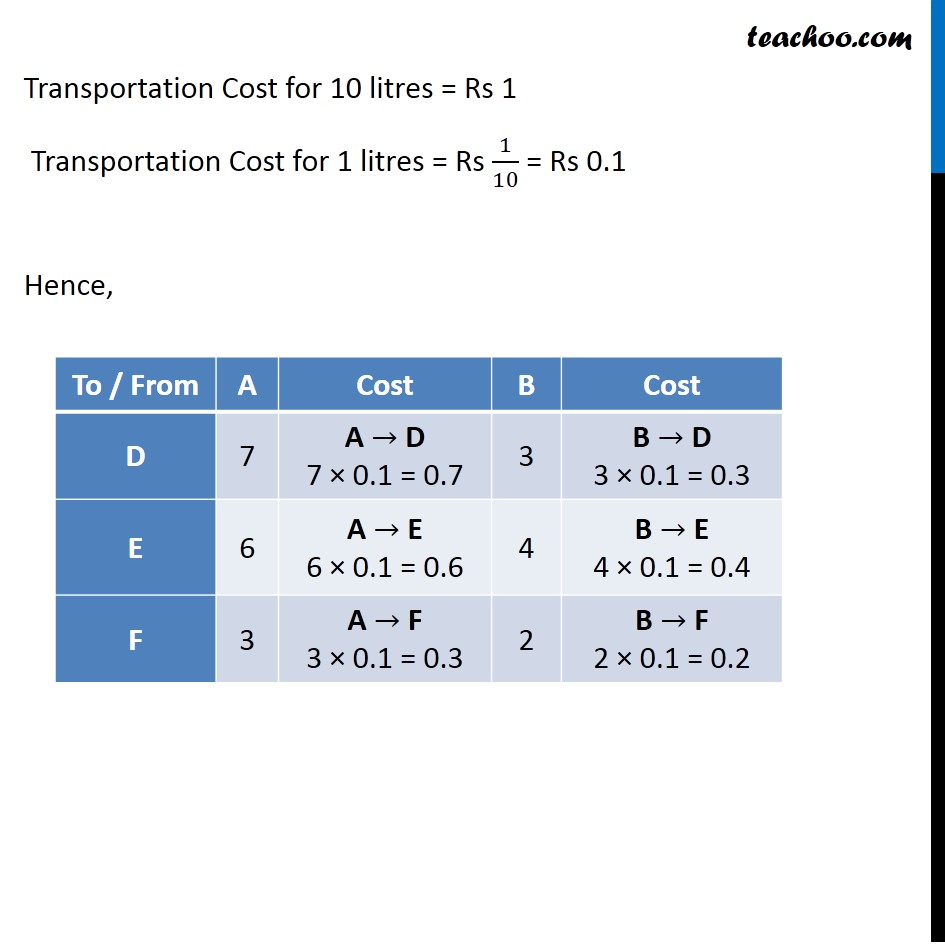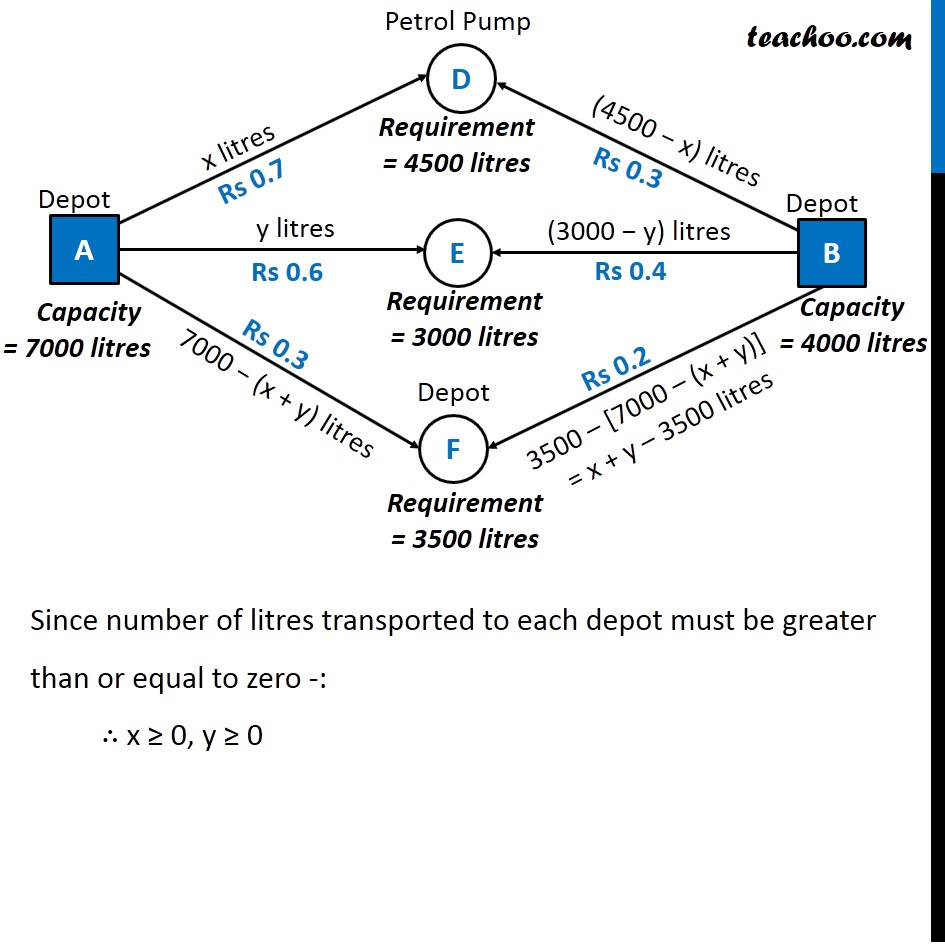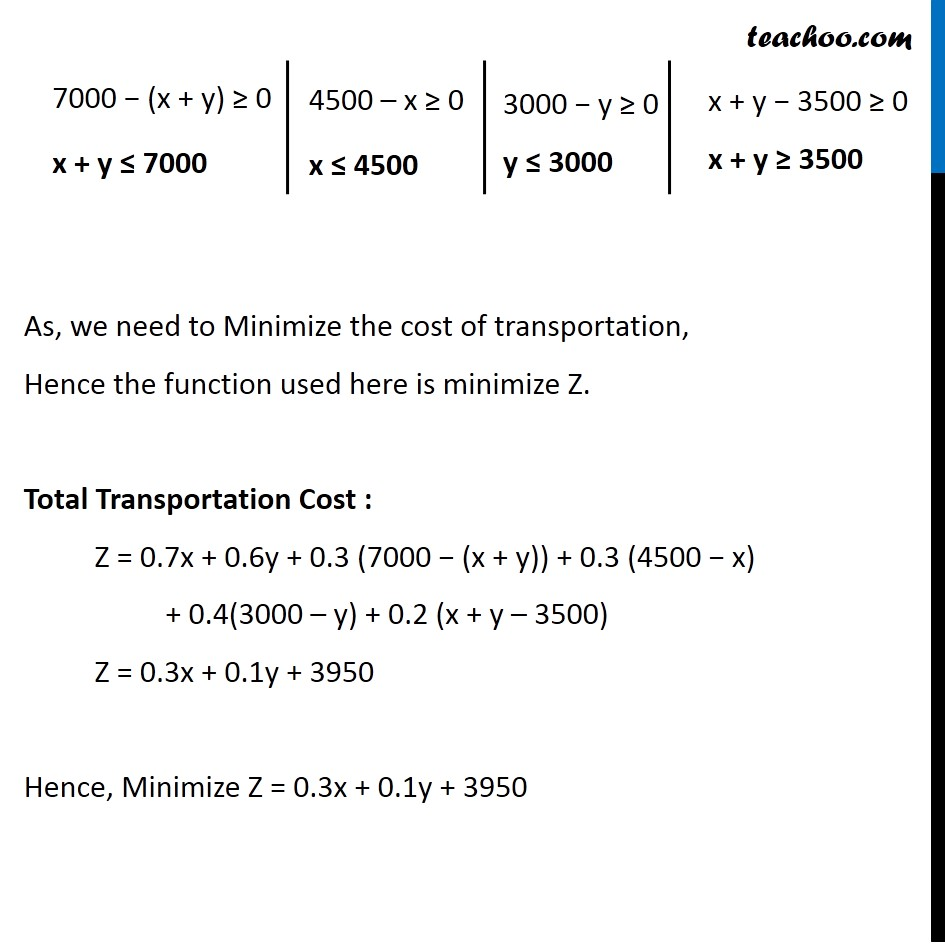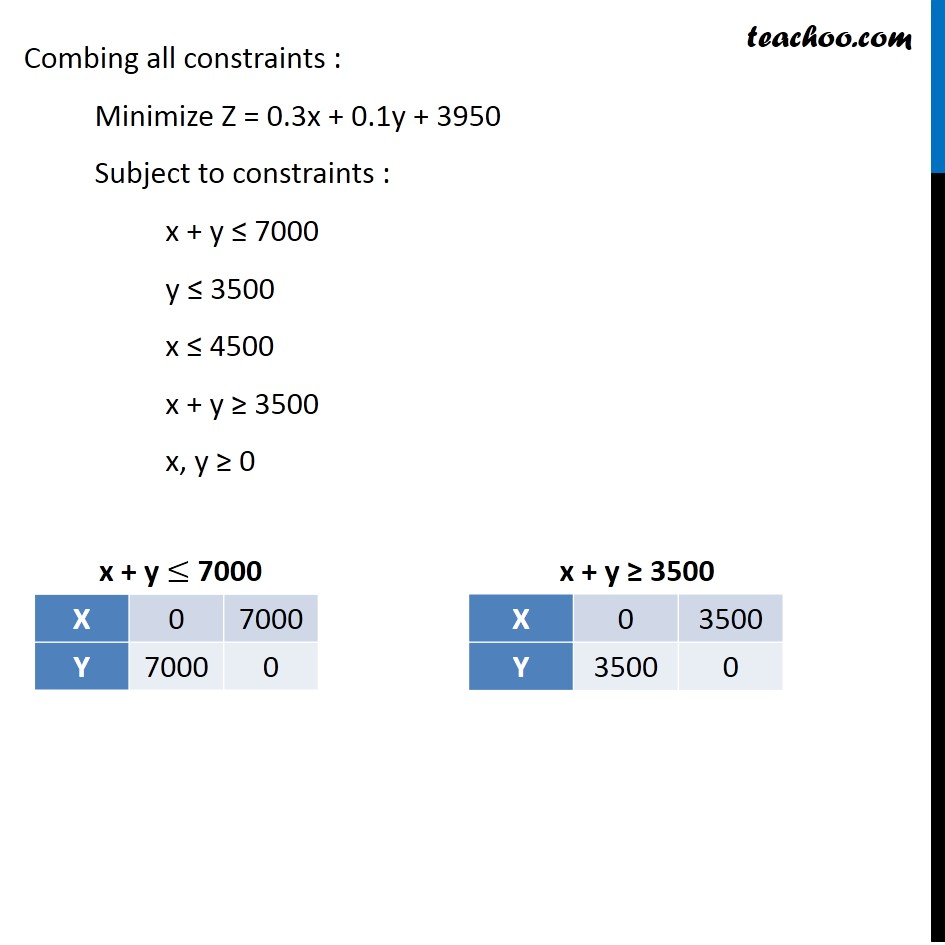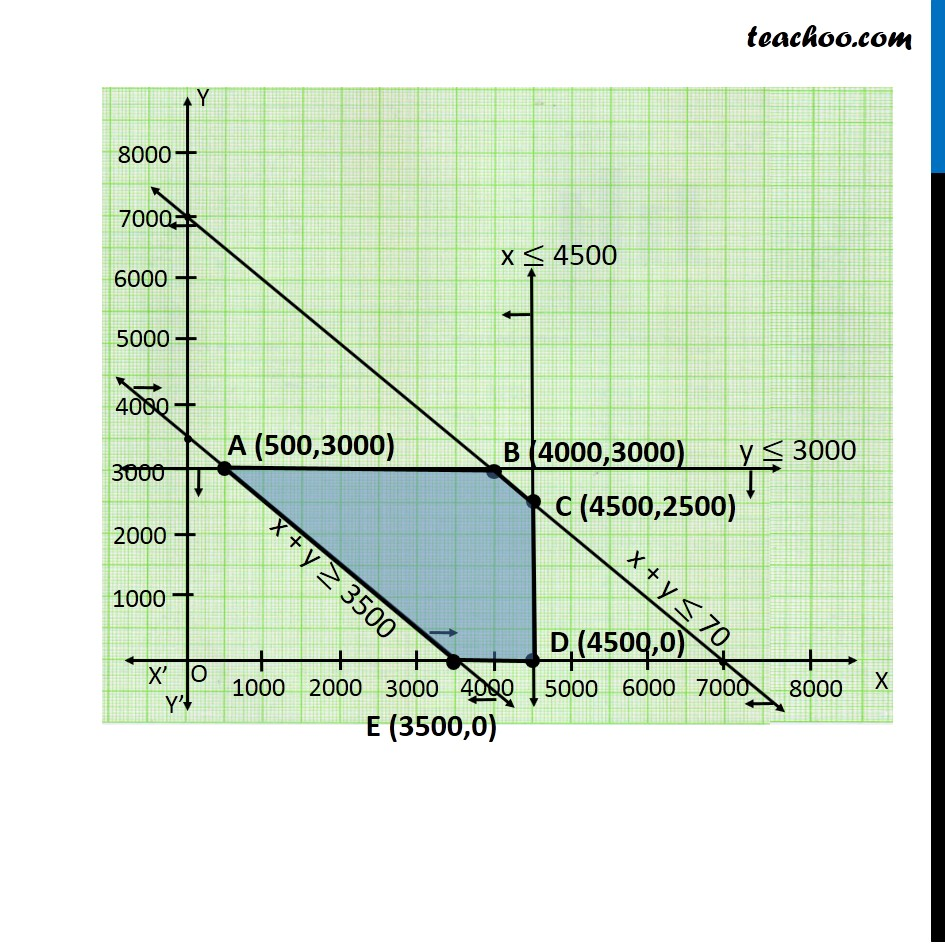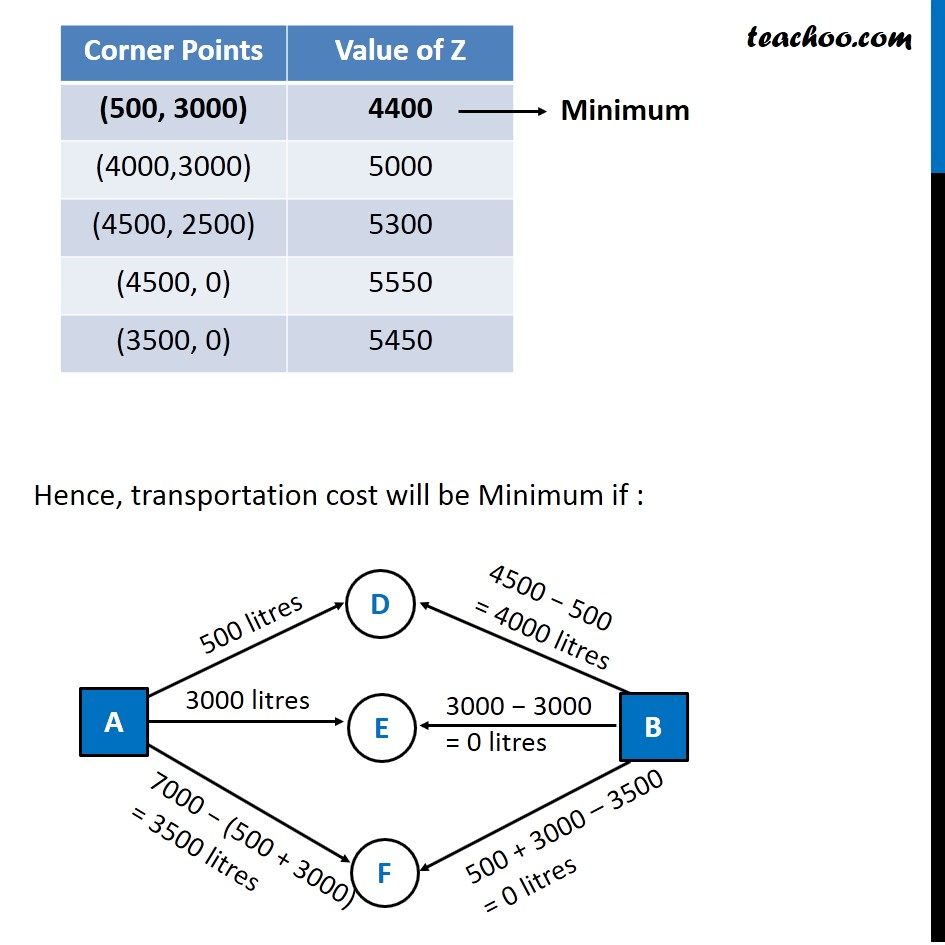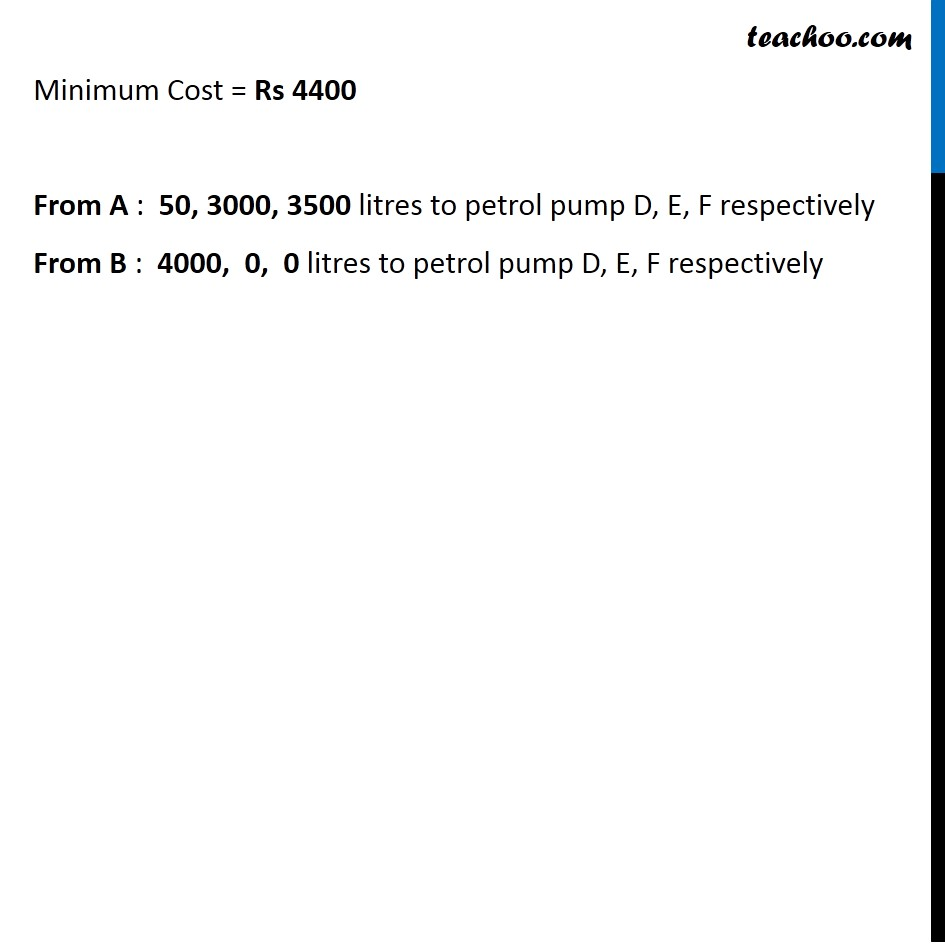Learn in your speed, with individual attention - Teachoo Maths 1-on-1 Class

### Transcript

Misc 7 An oil company has two depots A and B with capacities of 7000 L and 4000 L respectively. The company is to supply oil to three petrol pumps, D, E and F whose requirements are 4500L, 3000L and 3500L respectively. The distances (in km) between the depots and the petrol pumps is given in the following table: Assuming that the transportation cost of 10 litres of oil is Re 1 per km, how should the delivery be scheduled in order that the transportation cost is minimum? What is the minimum cost? Let depot A transport x litres to petrol pump D depot A transport y litres to petrol pump E Transportation Cost for 10 litres = Rs 1 Transportation Cost for 1 litres = Rs 1/10 = Rs 0.1 Hence, Since number of litres transported to each depot must be greater than or equal to zero -: ∴ x ≥ 0, y ≥ 0 7000 − (x + y) ≥ 0 x + y ≤ 7000 4500 – x ≥ 0 x ≤ 4500 3000 − y ≥ 0 y ≤ 3000 x + y − 3500 ≥ 0 x + y ≥ 3500 As, we need to Minimize the cost of transportation, Hence the function used here is minimize Z. Total Transportation Cost : Z = 0.7x + 0.6y + 0.3 (7000 − (x + y)) + 0.3 (4500 − x) + 0.4(3000 – y) + 0.2 (x + y – 3500) Z = 0.3x + 0.1y + 3950 Hence, Minimize Z = 0.3x + 0.1y + 3950 Combing all constraints : Minimize Z = 0.3x + 0.1y + 3950 Subject to constraints : x + y ≤ 7000 y ≤ 3500 x ≤ 4500 x + y ≥ 3500 x, y ≥ 0 Hence, transportation cost will be Minimum if : Minimum Cost = Rs 4400 From A : 50, 3000, 3500 litres to petrol pump D, E, F respectively From B : 4000, 0, 0 litres to petrol pump D, E, F respectively